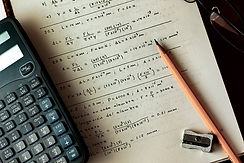## kS3 Math

The Math curriculum at SIH is presented in six content areas: Number, Algebra, Geometry, Measure, Handling data and Problem solving. The first five content areas are all underpinned by Problem
solving, which provides a structure for the application of mathematical skills. Mental strategies are also a key part of the Number content. This curriculum focuses on principles, patterns, systems, functions and relationships so that learners can apply their mathematical knowledge and develop a holistic understanding of the subject.## Our aims

At SIH the Math curriculum aims for students to:

• become fluent in the fundamentals of mathematics, including through varied and frequent practice with increasingly complex problems over time, so that pupils develop conceptual understanding and the ability to recall and apply knowledge rapidly and accurately.

• reason mathematically by following a line of enquiry, conjecturing relationships and generalisations, and developing an argument, justification or proof using mathematical language

• solve problems by applying their mathematics to a variety of routine and nonroutine problems with increasing sophistication, including breaking down problems into a series of simpler steps and persevering in seeking solutions.

## Find out more about the Keystage 5 curriculum. Years 12 and 13